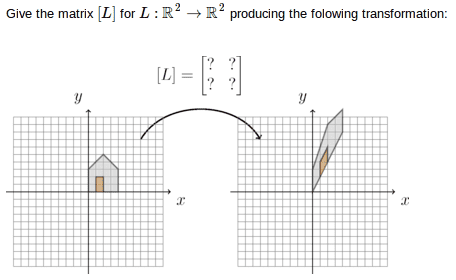# Linear Transformation/Mapping

## Homework Statement## Homework Equations

L(x,y) = L(x+y,x-y)
L(x,y) = L(x) + L(y)
L(kx) = kL(x)

## The Attempt at a Solution

I'm very confused on this. I've look all over and haven't figured this out yet. I'm there's one simple piece of info I'm missing and then it'll all make sense, but for the life of me I can't figure it out. The most I've come up with is showing what three points become.
L(0,3) = (0,3)
L(4,0) = (4,8)
L(4,3) = (4,11)

I don't know what to do from here.
Edit:
I need vectors, so would I turn my three points into vectors?
I think I need L(x)=[L]x=vx
Where v is the vector.

Last edited:

lanedance
Homework Helper
there are 4 useful points on the house that you should be able to use (excluding the oirgin which must map to itself as the map is linear), then consider L as a 2x2 matrix
$$L=\begin{pmatrix} a & b \\ c & d \end{pmatrix}$$

multplying L by an original point must give the transformed point for each of the points you have stated and teh one other, use these to solve for a,b,c&d

Last edited by a moderator:
So what I calculated:
L(0,3) = (0,3)
L(4,0) = (4,8)
L(4,3) = (4,11)
L(2,5) = (2,9)

L= ((a,c)|(b,d))

For the first point:
((a,c)|(b,d))(0,3) = (3b,3d)
3b= 0 -> b=0
3d = 3 -> d=1
Second point:
((a,c)|(b,d))(4,0) = (4a,4c)
4a = 4 -> a=1
4c = 8 -> c=2

So L = ((1,2)|(0,1))

lanedance
Homework Helper
ok looks good, the points with a zero in made life easy. Note the the action of the operator on two linearly independent vectors (equivalent to points here) whould be enough to solve

I would check with your 3rd or fourth point to make sure its consistent

ok looks good, the points with a zero in made life easy. Note the the action of the operator on two linearly independent vectors (equivalent to points here) whould be enough to solve

I would check with your 3rd or fourth point to make sure its consistent

Thank you, I did and everything checks out.